# Physics - Classical Mechanics - Exercises around Newtonian Gravity (part 2)

in StemSocial9 days ago (edited)[Image1]

## Introduction

Hey it's a me again @drifter1!

In this article we will continue with Physics, and more specifically the branch of "Classical Mechanics". Today's article is part two of Exercises on Newtonian Gravity. Part 1 can be found here.

So, without further ado, let's dive straight into it!

## Recap of Useful Formulas

The following formulas will be very useful for solving Problems around Gravity.

### Universal Law of Gravitation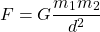where:
• d is the distance of the COMs of the two masses m1 and m2
• G is the Gravitation Constant which equals 6.674 × 10-11 N

### Weight or Force of Gravity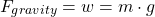### Gravitational Acceleration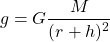where:
• r can be thought of as the median radius of a planet
• h as the height of an object in respect to the surface of the planet

### Gravitational Potential Energy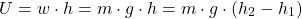or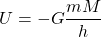ΔU = U2 - U1 is thus negative when the height decreases (h1 > h2).

### Gravitational Potential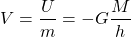## Two-Dimensional Problem's Field Acceleration

Let's start by calculating the Gravitational Acceleration g of the two-dimensional gravity problem that we solved during Part 1.

The total force aplied on mass mA = 4 Kg was calculated to be ΣF = 2.059 x 10-11.

Thus, the Gravitational acceleration g at the point where mA is in the field of the three other masses is: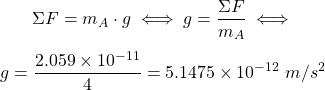## Gravitational Field on the Axis of a Ring (based on Ref1)

Let's consider the following gravitational field along the axis of a uniform ring: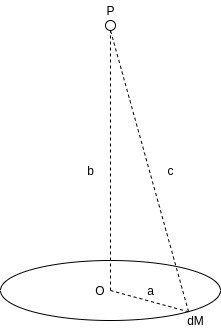[Custom Figure using draw.io]

The ring has a mass of M and a radius of a, and the points P are taken at a distance b along the axis of the ring. At the center of the ring the Gravitational field of course has a strengh of zero.

Let's find the maximum strength of the Gravitational Field along the axis of the ring in respect to the distance from the center O.

### Solution

In order to calculate the field of a ring, the ring has to be split into small masses dM, whose fields can then be summed up together.

The distance towards each of those small masses is equal to c, which can be easily calculated using Pythagoras's Theorem: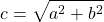The total gravitational field strengh dg of each of those masses dM is: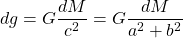Because each field dg points towards another direction a split of each vector into two components is necessary.

Doing that its easy to notice that there is another mass dM' that cancels out one of the components completely, which is something that happens for all masses dM: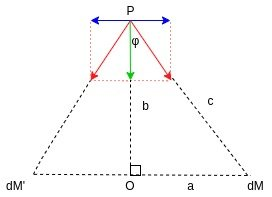[Custom Figure using draw.io]

Thus, only the component parallel to the axis of the ring will contribute towards the field strength.

Using the trigonometric function of cosinus, this component is defined as: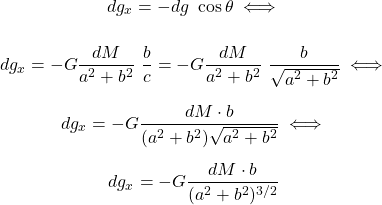Summing up the contributions of all those small masses dM, the total gravitational field g along the axis of the ring is calculated to be: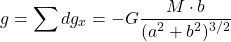The maximum value is reached at the point(s) where the derivative of g, g', is zero.

The derivative is equal to: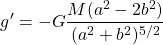The nominator is zero when: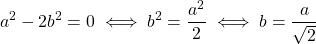Therefore, the maximum value is at a distance of b = a / √2, which gives us a field strength of: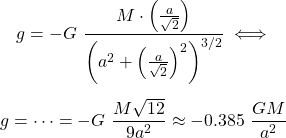The plot of the gravity field strength g in respect to the distance b, supposing that G = M = a = 1, looks as following: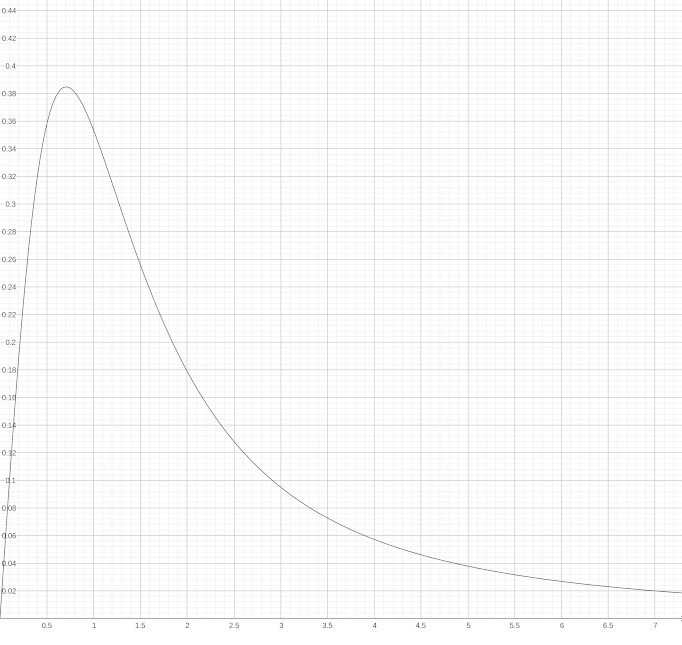[Custom Figure using GeoGebra]

## Speed of a Rollercoaster (Inversed Example 2 of Reference 2)

Consider a rollercoaster starts going down from an unknown height h and reaches a final speed of v = 25 m / s.

Find the value of h if friction is negligible and g = 9.8 m/s2.

### Solution

The initial velocity of the rollercoaster is zero (Kinitial = 0) and thus the rollercoaster initially has only gravitational potential energy.

The final potential energy is zero (Ufinal = 0), and thus, from Energy conservation, the initial potential energy equals the final kinetic energy, or mathematically: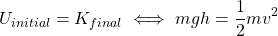The mass is cancelled out and the rest is known and thus the initial height h is: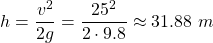## Final words | Next up

And this is actually it for today's post!

Next time we will get into a Physics explanation of the circular motion of Satellites...

See ya!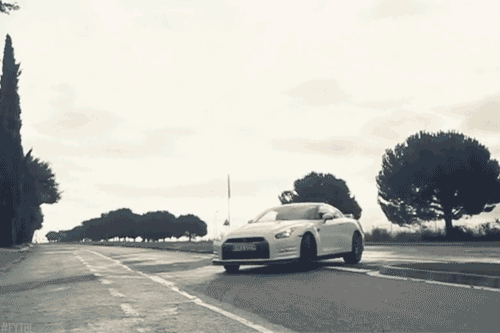Keep on drifting!
Sort:Thanks for your contribution to the STEMsocial community. Feel free to join us on discord to get to know the rest of us!

Please consider supporting our funding proposal, approving our witness (@stem.witness) or delegating to the @stemsocial account (for some ROI).

Please consider using the STEMsocial app app and including @stemsocial as a beneficiary to get a stronger support.

Congratulations @drifter1! You have completed the following achievement on the Hive blockchain and have been rewarded with new badge(s) :You published more than 450 posts. Your next target is to reach 500 posts.

If you no longer want to receive notifications, reply to this comment with the word `STOP`